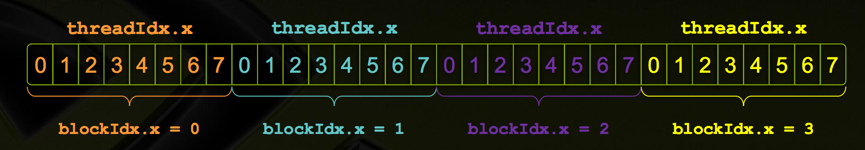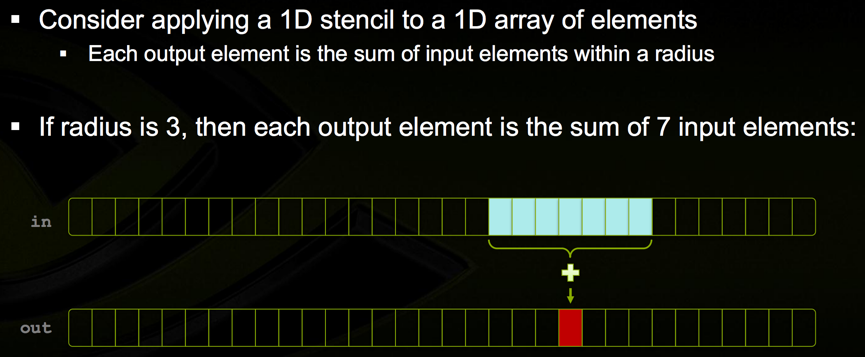# CUDA C/C++ Basics

Recently I tried to implement some rendering tasks and stucked by its very low speed. For acceleration CUDA is a very good tool.

Reference: http://www.nvidia.com/docs/IO/116711/sc11-cuda-c-basics.pdf

Topics:

• Heterogeneous Computing
• Blocks
• Indexing
• Shared memory
• Asynchronous operation
• Handling errors
• Managing devices

## 1 Heterogeneous Computing

Host(CPU+its memory) + Device(GPU+its memory)

1. Copy input data from CPU memory to GPU memory
2. Load GPU program and execute, caching data on chip for performance
3. Copy results from GPU memory to CPU memory

Example 1:

``````    __global__ void mykernel(void) {
}
int main(void) {
mykernel<<<1,1>>>();
printf("Hello World!\n");
return 0;
}
``````

__global__ indicates a function that runs on the device and is called by the host. «<»> marks a call from host code to device code, also called a “kernel launch”.

Example 2:

``````    __global__ void add(int *a, int *b, int *c) {
*c = *a + *b;
}

int main(void) {
int a, b, c; // host copies of a, b, c
int *d_a, *d_b, *d_c; // device copies of a, b, c
int size = sizeof(int);
// Allocate space for device copies of a, b, c
cudaMalloc((void **)&d_a, size);
cudaMalloc((void **)&d_b, size);
cudaMalloc((void **)&d_c, size);
// Setup input values
a = 2;
b = 7;
// Copy inputs to device
cudaMemcpy(d_a, &a, size, cudaMemcpyHostToDevice);
cudaMemcpy(d_b, &b, size, cudaMemcpyHostToDevice);
// Launch add() kernel on GPU
// Copy result back to host
cudaMemcpy(&c, d_c, size, cudaMemcpyDeviceToHost);
// Cleanup
cudaFree(d_a); cudaFree(d_b); cudaFree(d_c);
return 0;
}
``````

## 2 Blocks

add«< 1, 1 »>(); -> add«< N, 1 »>(); Each parallel invocation of add() is referred to as a block, the set of blocks is referred to as a grid. Each invocation can refer to its block index using blockIdx.x. blockIdx and threadIdx are 3D

``````    __global__ void add(int *a, int *b, int *c) {
c[blockIdx.x] = a[blockIdx.x] + b[blockIdx.x];
}

#define N 512
int main(void) {
int *a, *b, *c; // host copies of a, b, c
int *d_a, *d_b, *d_c; // device copies of a, b, c
int size = N * sizeof(int);
// Alloc space for device copies of a, b, c
cudaMalloc((void **)&d_a, size);
cudaMalloc((void **)&d_b, size);
cudaMalloc((void **)&d_c, size);
// Alloc space for host copies of a, b, c and setup input values
a = (int *)malloc(size); random_ints(a, N);
b = (int *)malloc(size); random_ints(b, N);
c = (int *)malloc(size);
// Copy inputs to device
cudaMemcpy(d_a, a, size, cudaMemcpyHostToDevice);
cudaMemcpy(d_b, b, size, cudaMemcpyHostToDevice);
// Launch add() kernel on GPU with N blocks
// Copy result back to host
cudaMemcpy(c, d_c, size, cudaMemcpyDeviceToHost);
// Cleanup
free(a); free(b); free(c);
cudaFree(d_a); cudaFree(d_b); cudaFree(d_c);
return 0;
}
``````

A block can be split into parallel threads

``````    __global__ void add(int *a, int *b, int *c) {
}

...
...
``````

## 4 Indexing

Combining Blocks and Threads:With M threads per block, a unique index for each thread is given by:

``````    int index = threadIdx.x + blockIdx.x * M;
``````

Use the built-in variable blockDim.x for threads per block：(gridDim.x is number of blocks per grid).

``````    int index = threadIdx.x + blockIdx.x * blockDim.x;
``````
``````    __global__ void add(int *a, int *b, int *c) {
int index = threadIdx.x + blockIdx.x * blockDim.x;
c[index] = a[index] + b[index];
}

#define N (2048*2048)
int main(void) {
int *a, *b, *c; // host copies of a, b, c
int *d_a, *d_b, *d_c; // device copies of a, b, c
int size = N * sizeof(int);
// Alloc space for device copies of a, b, c
cudaMalloc((void **)&d_a, size);
cudaMalloc((void **)&d_b, size);
cudaMalloc((void **)&d_c, size);
// Alloc space for host copies of a, b, c and setup input values
a = (int *)malloc(size); random_ints(a, N);
b = (int *)malloc(size); random_ints(b, N);
c = (int *)malloc(size);
// Copy inputs to device
cudaMemcpy(d_a, a, size, cudaMemcpyHostToDevice);
cudaMemcpy(d_b, b, size, cudaMemcpyHostToDevice);
// Launch add() kernel on GPU
// Copy result back to host
cudaMemcpy(c, d_c, size, cudaMemcpyDeviceToHost);
// Cleanup
free(a); free(b); free(c);
cudaFree(d_a); cudaFree(d_b); cudaFree(d_c);
return 0;
}
``````

Avoid accessing beyond the end of the arrays:

``````    __global__ void add(int *a, int *b, int *c, int n) {
int index = threadIdx.x + blockIdx.x * blockDim.x;
if (index < n)
a[index] = b[index] + c[index];
}

...
...
``````

Unlike parallel blocks, threads have mechanisms to efficiently communicate and synchronize.

## 5 Shared memory

Within a block, threads share data via shared memory.(Sometimes input elements are read by different threads) Extremely fast on-chip memory:

• By opposition to device memory, referred to as global memory
• Like a user-managed cache Declare using __shared__, allocated per block Data is not visible to threads in other blocks

Example:``````    __global__ void stencil_1d(int *in, int *out) {
__shared__ int temp[blockDim.x + 2 * RADIUS];
int gindex = threadIdx.x + blockIdx.x * blockDim.x;
// Read input elements into shared memory
temp[lindex] = in[gindex];
temp[lindex + blockDim.x] = in[gindex + blockDim.x];
}
// Apply the stencil
int result = 0;
result += temp[lindex + offset];
// Store the result
out[gindex] = result;
}
``````

Error here! Suppose thread 15 reads the halo before thread 0 has fetched it…(temp is shared) How to solve this? See ##6

``````    void __syncthreads();
``````

Synchronizes all threads within a block, used to prevent RAW / WAR / WAW hazards. All threads must reach the barrier. In conditional code, the condition must be uniform across the block.

Add `__syncthreads();` before Apply the stencil to ensure all the data are available.

## 7 Asynchronous operation

Kernel launches are asynchronous: Control returns to the CPU immediately.

## 8 Handling errors

All CUDA API calls return an error code cudaError_t Get the error code for the last error:

``````    cudaError_t cudaGetLastError(void)
``````

Get a string to describe the error:

``````    char *cudaGetErrorString(cudaError_t)
printf("%s\n", cudaGetErrorString(cudaGetLastError()));
``````

## 9 Managing devices

• Application can query and select GPUs
• Multiple host threads can share a device
• A single host thread can manage multiple devices
Written on July 29, 2018